# Quantizers and Quantization Noise¶

A quantizer is a signal processing block, that maps a continuous amplitude to a discrete amplitude. The output of the quantizer is discrete, meaning that it can only output $Q$ different values. Practically, the quantizer is an analog-to-digital converter, since it maps the continuous input amplitude to a digital representation of this value. Formally, the quantized output $Q[x]$ of some input value $x$, is given by

$$Q[x] = \arg \min_{l\in\mathcal{S}} |l-x|.$$

Here, the set $\mathcal{S}$ contains all possible output values of the quantizer, which we name quantization levels. What does that mean? It means, that for a given input $x$, the quantizer returns the quantization level $l$, which is closest to the input value. Hence, a quantizer is completely defined by its set of quantization levels $\mathcal{S}$.

Let's assume the quantizer should be able to quantize values between the input amplitudes $-U$ and $+U$, i.e. the peak-to-peak amplitude range equals $2U$. Furthermore, the quantization levels should be encoded by $b$ bits. This gives us a number of $2^q$ different quantization levels in $\mathcal{S}$. Then, a logical decision is to make the distance $\Delta_s$ between the quantization levels constant, and equal to

$$\Delta_s = \frac{2U}{q}.$$

Commonly, there are two different quantizers used, the mid-rise and the mid-tread quantizer. They differ in the setting of their levels: For the mid-tread we have $0\in\mathcal{S}$, whereas for the mid-rise quantizer we have $0\neq\mathcal{S}$, i.e. the mid-tread quantizer has a level for the zero output value. The quantization levels for both quantizers are defined by

\begin{align} \mathcal{S} &= \{-U+\frac{\Delta_s}{2}+\Delta_s\cdot(0,1,2,\dots, q-1)\}&\text{ for mid-rise}\\ \mathcal{S} &= \{-U+\Delta_s\cdot(0,1,2,\dots, q-1)\}&\text{ for mid-tread} \end{align}

Let us define some values for a demonstration of the quantizers:

b = 2            # number of bits for quantization.
q = 2**b         # 2 bits equal 4 quantization levels
U = 1            # max. Amplitude to be quantized
Delta_s = 2*U/q  # level distance

S_midrise = -U + Delta_s/2 + np.arange(q)*Delta_s


Further, let's define a function quantize that performs the quantization operation $$x_q(x) = \arg \min_{l\in\mathcal{S}} |l-x|^2.$$

def quantize(x, S):
X = x.reshape((-1,1))
S = S.reshape((1,-1))
dists = abs(X-S)

nearestIndex = dists.argmin(axis=1)
quantized = S.flat[nearestIndex]

return quantized.reshape(x.shape)


Let us draw the quantization curve of both quantizers to show their difference. The quantization curve is a diagram that shows the output of the quantizer for a range of inputs:Run code interactively  Hide
In = np.linspace(-1.3*U, 1.3*U, 2000)
Out_midrise = quantize(In, S_midrise)
plt.plot(In, Out_midrise, lw=2, label='mid-rise')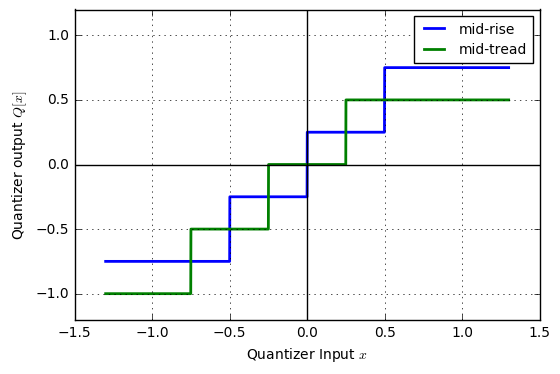As we can see, the mid-rise quantizer creates a symmetric distribution of quantization levels, whereas the mid-tread quantizer has more levels below zero than above zero. Note that since $2^b=q$ is an even number, the mid-tread quantizer cannot be symmetric in any case. In the following, we will focus on the mid-rise quantizer.

## Quantization Noise¶

The quantization operation introduces an error, because the infinite amount of different input amplitudes is mapped to a finite set of discrete quantization levels. The error between the quantizer's input and output is termed Quantization noise $n_q(t)$. When denoting with $s(t)$ the input signal and $s_q(t)$ the output signal of the quantizer, the following relation holds:

\begin{align} s_q(t)&=Q[s(t)]&&\text{Quantization operation}\\ n_q(t)&=s(t)-s_q(t)&&\text{Expression of quantization noise}\\ s_q(t)&=s(t)-n_q(t) \end{align}.

Looking at the last equation, the quantization noise can indeed be understood as a noise on top of the continuous-amplitude signal, hence its name. Let us create a sine wave and sent it through the quantizer and let's look at the quantized signal and the quantization noise.

First, we define a convinience function calcLevels which calculates the quantization levels for a given bit count b.

def calcLevels(U, b, quantization_type):
N_levels = 2**b
delta = 2*U / N_levels

if quantization_type == 'mid-rise':
levels = -U + delta/2 + np.arange(N_levels) * delta
levels = -U + np.arange(N_levels) * delta
else:
raise RuntimeError("Unknown quantization type!")

return levels


Now, let's generate a sine signal and show the resulting quantization noise.

Fs = 100  # the sampling frequency
f = 1     # frequency of the sine wave
T = 3     # time duration to look at
t = np.arange(0, T, 1/Fs)
s = np.sin(2*np.pi*f*t)
def showQuantizationNoise(b):
S = calcLevels(U, b, 'mid-rise')
s_q = quantize(s, S)
n_q = s - s_q

plt.plot(t, s, label='$s(t)$')
plt.plot(t, s_q, lw=2, label='$s_q(t)$')
plt.plot(t, n_q, label='$n_q(t)$');
plt.text(0.25, -0.5, 'b=%d' % b, ha='center', bbox=dict(facecolor='white'))Run code interactively  Hide



As you see, with increasing number of quantization bits, the quantization error almost vanishes. However, an important measure is the signal-to-quantization noise ratio (SNR), and we are going to measure it. With high enough bit counts, the quantization noise is approximately uniformly distributed within $\pm\frac{\Delta_s}{2}$. In literature, e.g. Proakis: Digital Signal Processing, it has been shown that the quantization SNR for a sine-wave with full excitation (i.e. the sine amplitude equals the quantization range) can be given by

$$SNR\approx (1.76 + 6.02b)dB,$$

with $b$ being the number of bits for the quantization.

Let us write a function to measure this quantization noise. Here, we directly convert the SNR to dB scale.

def calcQuantizationNoise(signal, b):
S = calcLevels(U, b, 'mid-rise')
signal_q = quantize(signal, S)
n_q = signal - signal_q

P_S = (signal**2).sum()
P_N = (n_q**2).sum()

SNR = 10*np.log10(P_S/P_N)  # use
return SNR


We can now plot the quantization noise against the number of bits and compare it against the theoretical curve of $1.76+6.02b$.

bits = np.arange(1, 17)
SNR = [calcQuantizationNoise(np.sin(2*np.pi*t*f), b) for b in bits]
plt.plot(bits, SNR, 'o', label='SNR measured')
plt.plot(bits, 1.76+6.02*bits, 'k--', lw=2, label='$1.76+6.02b$')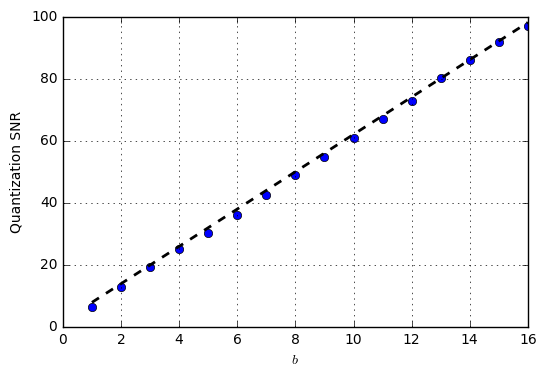As we see, the approximation holds very well. Let us now look at what happens when we decrease the amplitude of the sine:

for A in [1, 0.5, 0.1]:
SNR = [calcQuantizationNoise(A*np.sin(2*np.pi*t*f), b) for b in bits]
plt.plot(bits, SNR, 'o-', label=r'SNR measured, $s(t)=%.1f \sin(2\pi ft)$' % A)

plt.plot(bits, 1.76+6.02*bits, 'k--', lw=2,label='$1.76+6.02b$')
plt.legend(fontsize=10, loc='lower right')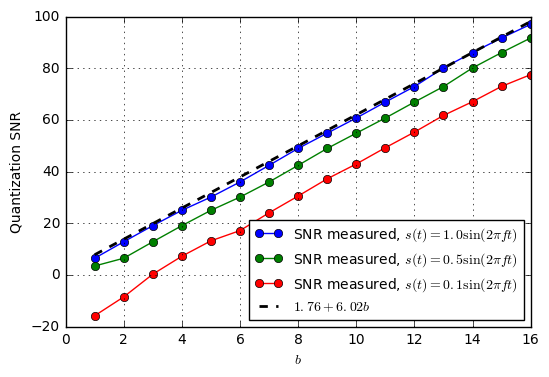As we see, the quantization SNR degrades when the sine amplitude decreases. This is reasonable, since the quantization noise only depends on the number of quantization bits $b$, and is hence independent of the signal amplitude and so is the quantization noise power. On the other hand, the signal power decreases, when the amplitude decreases. Hence, we see a SNR degradation. However, the important notice is that the improvement in SNR for each additional bit remains to be $6$dB, independent of the signal amplitude.

Let us now measure the quantization SNR for a signal that is just Gaussian noise:

signal = np.random.randn(len(t))  # generate some random signal

plt.subplot(121)
SNR_noise = [calcQuantizationNoise(signal, b) for b in bits]
plt.plot(bits, SNR_noise, 'o-', label=r'SNR measured, $s(t)=%.1f \sin(2\pi ft)$' % A)
plt.plot(bits, 1.76+6.02*bits, 'k--', lw=2,label='$1.76+6.02b$')

plt.subplot(122)
signal_q = quantize(signal, calcLevels(U, 3, 'mid-rise'))
plt.plot(t, signal, label='$s(t)$')
plt.plot(t, signal_q, label='$s_q(t)$')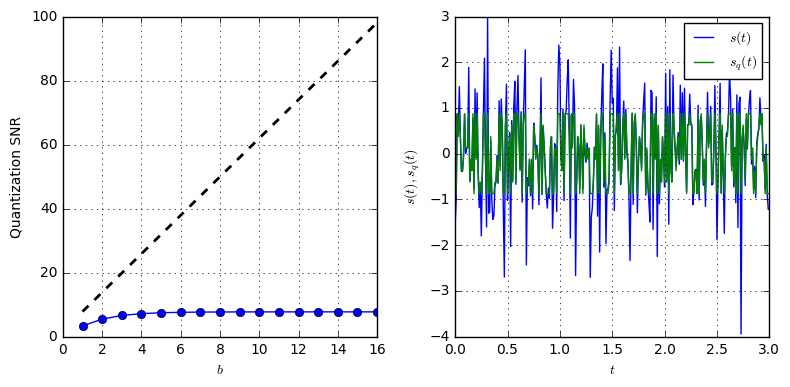What's going on here? The quantization SNR is pretty low despite 14 bits resolution? Looking at the original and quantized signal reveals the problem: The quantizer is simply in overdrive: The input signal is higher than the quantizer can output. So, it can never reach a good quantization. Let us eventually reduce the noise amplitude and see if this helps:

signal = 0.1 * np.random.randn(len(t))

plt.subplot(121)
SNR_noise = [calcQuantizationNoise(signal, b) for b in bits]
plt.plot(bits, SNR_noise, 'o-', label=r'SNR measured, $s(t)=%.1f \sin(2\pi ft)$' % A)
plt.plot(bits, 1.76+6.02*bits, 'k--', lw=2,label='$1.76+6.02b$')

plt.subplot(122)
signal_q = quantize(signal, calcLevels(U, 3, 'mid-rise'))
plt.plot(t, signal, label='$s(t)$')
plt.plot(t, signal_q, label='$s_q(t)$')
#plt.xlim((0,0.3))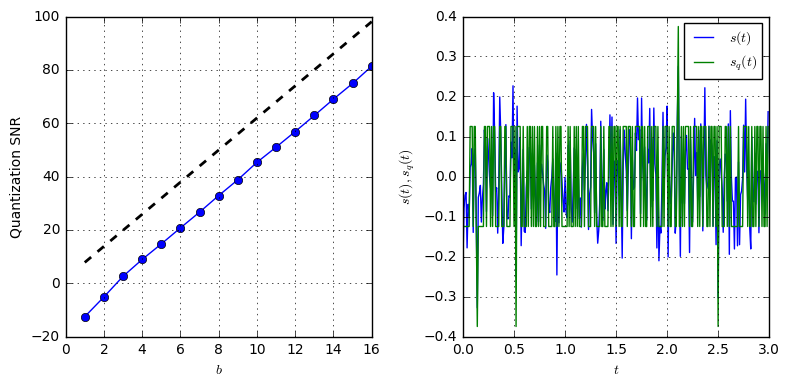Now we see that the quantization SNR increases with 6dB for each bit. But, looking at the signals, we see another important thing: Even though the quantizer would be able to output values between $\pm 1$, most of its values are concentrated with in $\pm 0.1$. This is due to the character of the Gaussian noise signal which concentrates mostly around $s(t)=0$. Hence, to improve the SNR it would make sense to increase the resolution in the lower quantization levels at the cost of a coarser discretization in the higher amplitudes. This technique is known as non-linear quantizers but is out of scope here.

## Summary¶

• A quantizer maps a continuous analog amplitude to a discrete amplitude level.
• A quantizer introduces quantization noise, which can indeed be treated as extra noise in the system, as long as the quantizer resolution is high enough.
• The quantization noise reduces by $6$dB for each additional quantization bit. This number is independent of the quantization excitation or signal type.Do you have questions or comments? Let's dicuss below!

### Related posts

DSPIllustrations.com is a participant in the Amazon Services LLC Associates Program, an affiliate advertising program designed to provide a means for sites to earn advertising fees by advertising and linking to amazon.com, amazon.de, amazon.co.uk, amazon.it.